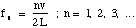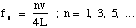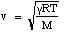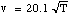Swarthmore College

Engineering Methodology

Resonance in 1D

In lecture this week, you learned about resonance phenomena and regenerative feedback, two central concepts in musical acoustics. The Matlab assignment below asks you to generate new sounds with user-definable characteristics, based upon these principles.

Problem

A gas-filled pipe of uniform cross section and length L can maintain acoustic standing waves at frequencies given by:(open-open)

or(open-closed)

where n is the harmonic number and v is the sound speed in the gas given by:where g is the ratio of specific heats for the gas, R is the universal gas constant, M is the molecular weight of the gas, v is in m/s and T is the absolute temperature in Kelvin. Putting in the constants for air (g = 1.4, R = 8.31, and M = 2.88¥10-2) yields.

The relative amplitudes of the standing wave frequencies in the vibration recipe of the total sound is complicated, and depends upon such factors as the frequency dependence of the end correction and the means by which the sound is generated.

Write a Matlab script to prompt the user for the air temperature (convert from °F to K), the length of the tube L (convert from ft. to m if you wish), and whether the tube is open-open or open-closed. For this last part, you'll have to make use of the special form of the Matlab input command that allows string inputs such as h = input('your text here', 's') and the if statement. The program should then ask the user for the number of harmonics N to use in building the sound, and for the amplitude of each harmonic relative to the fundamental. Using a FOR loop, the program should add up the harmonics one by one according to the user's recipe (starting from the fundamental) and play (using the Matlab sound function) the sound of the instrument so the user can hear it.

Using the drop box on the Classes file server, hand in your script only (with your username followed by the number 7 as the script name before the ".m").

Challenge assignment (only if you find the assignment above unchallenging)

For each time through the loop, the program should display a double graph of each added harmonic as well as their sum: on the top a plot of sound pressure and on the bottom a plot of particle velocity with normalized amplitude on the vertical axis and distance along the pipe on the horizontal axis (up to the length of the tube L).

Matlab solution